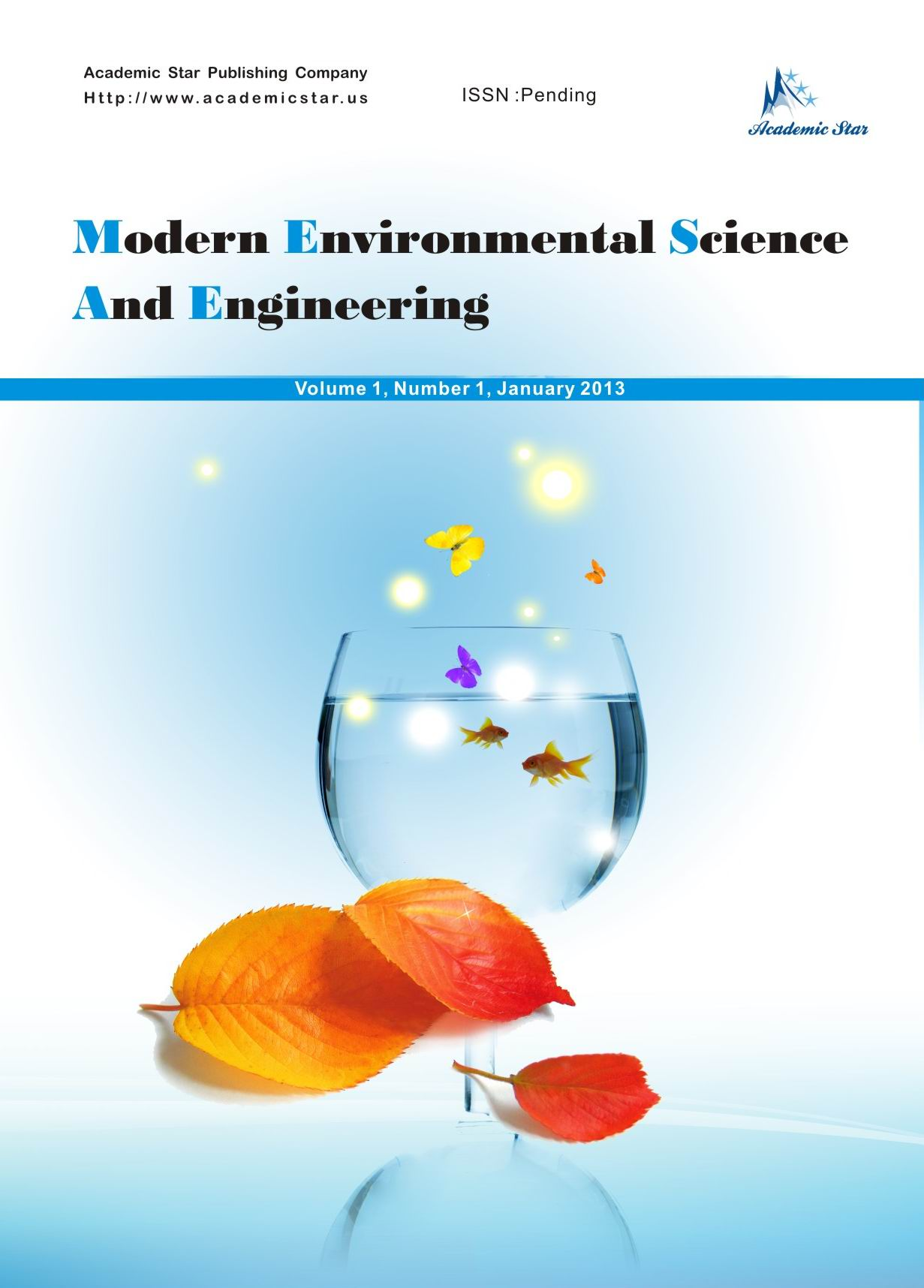Technology and Engineering• ISSN: 2333-2581
• Modern Environmental Science and Engineering

# Analysis of Sunshine Duration Data Using Two-Parameter Weibull Distributions

M. Bashahu, and D. Ntirandekura

Department of Physics and Technology, Institute of Applied Pedagogy, University of Burundi, Burundi

Abstract: Previous studies have shown that beta probability density functions (β-PDFs) are very good fits of the observed relative frequency distributions of data from various stations for dimensionless physical quantities such as the clearness index (kt), the diffuse fraction (kd ), the relative wind speed (vr) and the relative sunshine duration (sr). In this work, attempts have been made to fit actual relative frequency distributions of sunshine duration (S) data with 2-parameter Weibull PDFs, which are otherwise often used to fit experimental frequency distributions of wind speed and wind power density data. The monthly and annual observed relative frequency distributions (fi,obs) of S data from a 5-years period of records at six Burundian stations have been implemented and their main characteristics have been derived. The shape and scale parameters (k and c, respectively) of the theoretical (Weibull) PDFs (fi,th) fitting those distributions (fi,obs) have been determined by the means of the graphical method. The validity of the obtained formulations has been checked through three complementary statistical tests, namely the mean bias error (MBE), the root mean square error (RMSE) and the t-statistics. For any of the 78 S datasets, the MBE and the RMSE have been found very low as expected, since they lie in the ranges [-0.01;+0.009] and [0.040;0.052], respectively. Moreover, the values of t were lower than their critical counterparts (tc). Those results show that the 2-parameter Weibull PDFs obtained so far are not statistically different from the related observed relative frequency distributions of S data. They should be therefore used as input data when planning solar energy systems for the stations of this study.

Key words: relative frequency distributions, statistical tests, sunshine duration data, 2-parameter Weibull PDFs